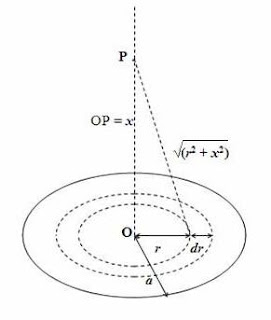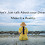## Pages

`“Life is like riding a bicycle.  To keep your balance you must keep moving.”–Albert Einstein`

## Saturday, January 14, 2012

### AP Physics C - Answer to Free Response Practice Question on Electrostaics

"I am a friend of Plato, I am a friend of Aristotle, but truth is my greater friend".
–Sir Isaac Newton

In the post dated 11th January 2012, a free-response question for practice was given to you. As promised, I give below a model answer. The question also is given for convenience:A thin circular nonconducting disc of radius ‘a’ resting horizontally on the ground has positive charges sprayed on its top surface so that it has a uniform surface charge density σ. The centre of the disc is O and the axis of the disc is vertical (Fig.). There are no other electric charges in the vicinity for producing any appreciable interaction on the charges on the disc and the acceleration ue to gravity at the place is g. Now answer the following questions:

(a) Derive an expression for the electric potential at a point on the axis of the disc at distance x from its centre.

(b) What is the electric potential at the centre of the disc?

(c) A positively charged particle of mass m and charge q with specific charge (q/m) of 8ε0g/σ is released from rest from a point P on the axis of the disc. The distance of point P from the centre of the disc is d. If the particle just reaches the centre O of the disc, determine the value of d.

(d) Explain qualitatively the nature of motion of the charged particle after its release from the point P stated in part (c) above.

[This question was asked in a different form in IIT entrance examination earlier].

(a) The disc can be imagine to be made of a large number of concentric rings of radii ranging from zero to a. To derive an expression for the potential at a point P (Fig.) on the axis of the disc at distance x from the centre, consider one such ring of radius r and width dr as shown in the figure. The total charge on the ring is rdrσ since the area of the top surfaceof the ring is rdr.

All charges on the ring are at the same distance √(r2 + x2) from the point P so that the electric potential at P due to the charges on the ring is (1/4πε0){rdrσ/√(r2 + x2)} where ε0 is the permittivity of free space.

The electric potential at P due to the charges on the entire disc [(P)] is obtained by adding the contributions of all the rings forming the entire disc. Therefore we have

V(P) = 0a [(1/4πε0){rdrσ/√(r2 + x2)}]dr

Or, V(P) = (σ/2ε0) 0a [r/√(r2 + x2)] dr

Since the value of the integral is √(r2 + x2) and the upper and lower limits (of r) are a and zero, we get

V(P) = (σ/2ε0)[√(a2 + x2) x]

(b) The electric potential at the centre of the disc [V(O)] is obtained by putting x = 0 in the above general expression for the potential on the axis.

Therefore, V(O) = σa/2ε0

(c) When the particle falls from height d and just comes to rest (momentarily) at O, as is evident from the question, it gains electric potential energy at the cost of gravitational potential energy. Therefore, by the law of conservation of energy we have

mgd =q[V(O) V(P)] where V(P) here is the electric potential energy when the point P is at height d and is equal to (σ/2ε0){√(a2 + d2) d}. Therefore we have

mgd =q[(σa/2ε0) – (σ/2ε0){√(a2 + d2) d}]

Or, mgd =(qσ/2ε0) [a –{√(a2 + d2) d}]

Therefore, d =(q/m)(σ/2ε0g) [a –{√(a2 + d2) d}]

Since the specific charge q/m =8ε0g/σ, as given in the question, we get

d =(8ε0g/σ)(σ/2ε0g) [a –{√(a2 + d2) d}] = 4[a –{√(a2 + d2) d}]

Or, 4√(a2 + d2) = 3d + 4a

Squaring, 16a2 + 16d2 = 9d2 + 16a2 + 24ad

Or, 7d2 = 24ad from which d = 24a/7

(d) Initially the particle moves down with an acceleration because of the gravitational pull mg even though the electrostatic repulsive force due to the like charges on the disc opposes the motion. As the particle approaches the disc, the electric force increases where as the gravitational force remains constant. But the particle overshoots its final equilibrium position because of inertia. The downward motion of the particle gets decelerated because of the increasing electric repulsive force and it comes to rest momentarily at the central point O. The particle then moves upwards because of the electric repulsive force which is greater in magnitude here, compared to the gravitational pull. The particle oscillates about its final equilibrium position and finally comes to rest at the final equilibrium position which is at a height from the point O.

#### 1 comment:

1.Very informative and cute and simple in design. The font and etc are good. The quotations are used very nicely. Thank you for sharing. Please visit my blog